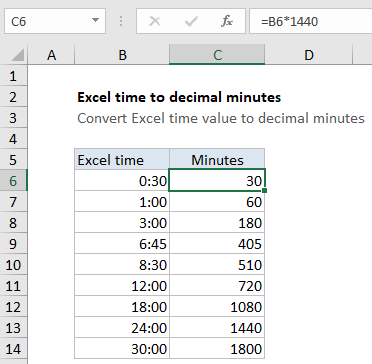# Convert Excel time to decimal minutes

This tutorial shows how to Convert Excel time to decimal minutes using example below.

To convert a valid Excel time into decimal minutes, you can multiply by 1440.

## Formula

`=A1*1440`## Explanation

In the example shown, the formula in C6 is:

`=B6*1440`

which returns a value of 30.

### How this formula works

In the Excel time system, one 24-hour day is equal to 1. This means times and hours are fractional values of 1, as shown in the table below:

Hours Time Fraction Value
1 1:00 AM 1/24 0.04167
3 3:00 AM 3/24 0.125
6 6:00 AM 6/24 0.25
4 4:00 AM 4/24 0.167
8 8:00 AM 8/24 0.333
12 12:00 PM 12/24 0.5
18 6:00 PM 18/24 0.75
21 9:00 PM 21/24 0.875

Because each hour can be represented as 1/24,  you can convert an Excel time into decimal hours by multiplying the value by 24, and convert to decimal minutes by multiplying the value by 1440 (24 * 60) . With the time value 6:00 cell A1, you can visualize the conversion like this:

```=A1*(24*60)
=(6/24)*1440
=0.25*1440
=360```

The Excel time 6:00 converts to 360 minutes.